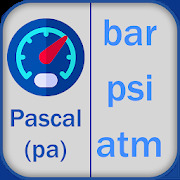#### Alphabetical

A | B | C | D | E | F | G | H | I | J | K | L | M | N | O | P | Q | R | S | T | U | V | W | X | Y | Z

# Pressure Converter Equation and CalculatorEasy to converter pressure unit.<br><br>Pressure is an expression of force exerted on a surface per unit area. The standard unit of pressure is the pascal (Pa),equivalent to one newton per meter squared (N/m 2 or N · m -2). <br>Alternatively, pressure can be measured in dynes per centimeter squared (dyn/cm 2 or dyn · cm -2).<br>To convert from pascals to dynes per centimeter squared, multiply by 10. Conversely, multiply by 0.1.<br><br><br>In this App unit conversions pascal (Pa) megapascal (MPa) kilopascal (kPa) hectopascal (hPa) decipascal (dPa) And Much More.